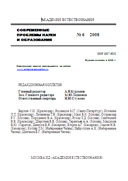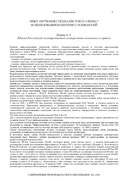Приложение к журналу «Современные проблемы науки и образования» ISSN - 1817-6321

 PDF-версия статьиOPTIMAL VALUES OF PPM PARAMETER H

Siberian Federal University, Krasnoyarsk, Russia e-mail: multypha@mail.ru

The application of PPM allows increasing the reliability (strictly speaking, fault tolerance) of the multiphase induction motor drive in spite of the fact that the number of wires between the inverter and linear motor is increased when the phase number m is extended.

The optimal value Ho of PPM parameter H for the given phase number m was found by the authors of this paper. When the equation H=Ho is obtained, both the drive system mass-and-overall dimensions decrease and its reliability (i.e. fault tolerance) increase are achieved.

The value Ho is determined as follows:

1) Ho=[Ah] if {Ah}<=0.5;

2) Ho=[Ah+1.0] if {Ah}>0.5,

where

Ah=[(Ka^2+4*Ka*Hm)-Ka]/2

or

Ah=-[(Ka^2+4*Ka*Hm)+Ka]/2,

where [Ah] is the integer part of the number Ah, {Ah} is the fractional part of the number Ah, Hm is the maximal value of parameter H for the given value of phase number m, Hm =[Dm], [Dm] is the integer part of the number Dm=m/3.

The parameter Ka shows in how many times the decrease in drive mass-and-overall dimensions is more important than the increase of its survivability for the drive system developer (i.e. the parameter Ka establishes the compromise between the above-mentioned mass-and-overall dimensions decrease and the linear drive survivability increase).

The values of the parameter Ho are given in [1-3] for 2<=Hm<=10 and some set of the parameter Ka values.

For example, at the previous design stage the developer has chosen value of phase number m=30 (in this case Hm=30/3=10). If he has taken an interest in both the motor mass-and-overall dimensions minimization and its survivability enlargement to the same extent, he chooses the value Ka=1.0. It follows from Table 3 that in this case the value of the parameter Ho=3.

In a number of cases (for example, in the field of oil deep-well pumps) it is especially important to obtain at once the linear drive mass-and-overall dimensions decrease and its survivability enlargement.

REFERENCES:

1. Brazhnikov A.V., and Belozerov I.R., "Over-Phase Control of Inverter Multiphase AC Linear Drives", Proceedings of 8th International Symposium on Linear Drives for Industry Applications LDIA‘2011, Eindhoven University of Technology, Eindhoven, the Netherlands, July 3-6, 2011, CD-ROM, paper No 172, 6 p.

2. Brazhnikov A.V., and Belozerov I.R., "Non-Traditional Control and Advantages of Multiphase AC Inverter Drives", Proceedings of IEEE International Conference on Energy, Automation and Signal "ICEAS ‘2011", Bhubaneswar, Orissa, India, December 28-30, 2011, pp. 781-786.

3. Brazhnikov A.V., and Belozerov I.R., "Over-Phase Control of Inverter Multiphase AC Linear Drives", Journal "Mechatronics", Elsevier Publishing Company, DOI 10.1016/j.mechatronics.2012.02.003 (Corrected Proof, Available online since March 5, 2012), in press.

ОПУБЛИКОВАНО

Brazhnikov A.V. OPTIMAL VALUES OF PPM PARAMETER H. // Современные проблемы науки и образования - 2012.-№6. (приложение "Технические науки"). - C. 24